# Twisted torus

That plot is from my German blog TikZ.de.

I planned to model this nice cake:(Foto by Guido Draheim).

Like a cruller, just, ehm, more digital and mathematical.

How do we draw it?

Let’s think of a cross-section. In polar coordinates the sine function sin(x) gives a circle, sin(3x) are three leaves, we add some radius (1,25) as a middle piece. That gives us the function sin(3x) + 1.25 in polar coordinates:We embed it in the three-dimensional space, like in the xy plane with z=0 as (x,y,z)(t) = ( cos(t)(sin(3t)+1.25), sin(t)(sin(3t) + 1.25), 0 ):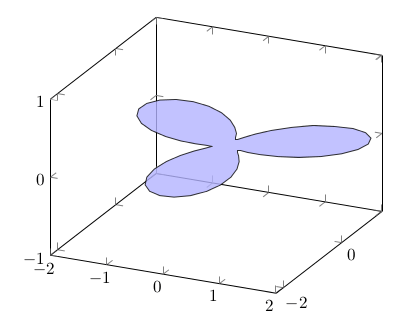Or rotated a bit: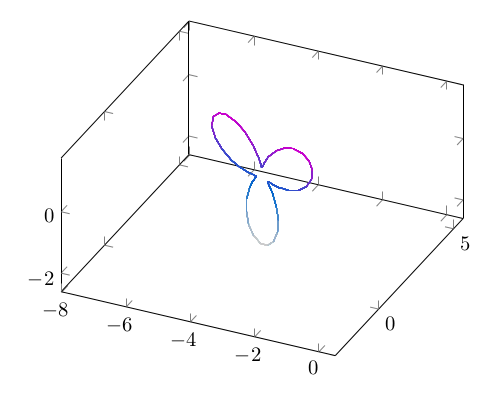We can move it in the space by drawing in the xz plane and move with linear y: (x,y,z)(t) = ( cos(t)(sin(3t)+1.25), t, sin(t)(sin(3t)+1.25) ). That becomes: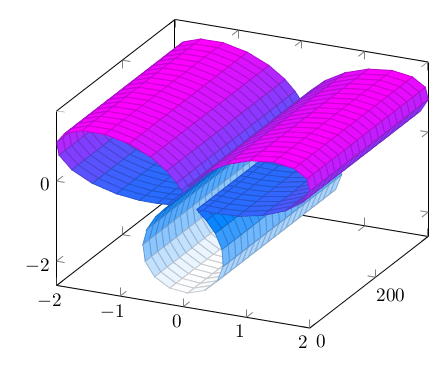But we want to rotate it. For doing this, we use a torus function , like this one:

x(t,s) = (2+cos(t))cos(s+pi/2)
y(t,s) = (2+cos(t))sin(s+pi/2)
z(t,s) = sin(t)

We combine it with our function:

x(t,s) = (6+(sin(3t)+1.25)cos(t))cos(s)
y(t,s) = (6+(sin(3t)+1.25)cos(t))sin(s)
z(t,s) = (sin(3t)+1.25)sin(t)

Here is a cut, half circle rotation: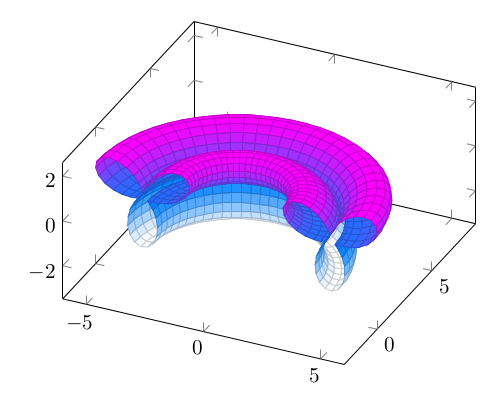Here is the whole torus based rotated 3d image of the original function: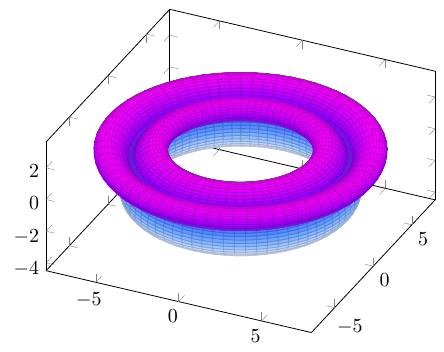And now to the twist. We can twist it by adding a multiple of t or y in the function argument and achieve the rotation with growing y:Math done, let’s add color. Ok, and now the code:

% !TEX lualatex
% Mit LuaLaTeX übersetzen, weil die Berechnungen zu aufwendig für pdfLaTeX sind
\documentclass{standalone}
\usepackage{pgfplots}
\usetikzlibrary{backgrounds}
\begin{document}
\begin{tikzpicture}
\begin{axis}[axis equal,
hide axis,
/tikz/background rectangle/.style = {
left color    = black,
right color   = black!20,
},
show background rectangle
]
surf,
miter limit = 1,
domain      = 0:360,
y domain    = 0:360,
samples     = 60,% low res for online compiler, take 100 for the image below,
samples y   = 40,% % low res for online compiler, take 70 for the image below,
z buffer    = sort,
colormap/hot2,
]
( {(6+(sin(3*(x+3*y))+1.25)*cos(x))*cos(y)},
{(6+(sin(3*(x+3*y))+1.25)*cos(x))*sin(y)},
{((sin(3*(x+3*y))+1.25)*sin(x))} );
\end{axis}
\end{tikzpicture}
\end{document}

With more resolution, samples=100 and samples y=70:All codes and explanation are also here: